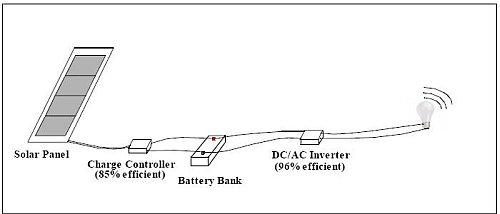# Calculating Solar Power Needs - How to Figure Out Your Energy Needs

Watts, Amps, Voltages? What does it all mean? Calculating solar power needs is not hard once you understand the basics. First you need to know how much energy you require, and from there you can figure out the rest of the system. In this example, we'll use a 16 W compact florescent light bulb running on AC current. Our calculations will start at the light bulb and will work backwards to the solar panels.

This article may contain affiliate links. When you purchase through links on this site, I may earn a small commission at no extra cost to you.## How Much Energy Do Your Appliances Use?

Power consumption is recorded in Watts. For instance, there are 16 W, 60 W and 100 W light bulbs. The higher the rating, the more energy it consumes. If you have a 100 W light bulb turned on for 1 hour, it will consume 0.1 kWH:

100 W x 1 Hour / (1000 W / 1 kW) = 0.1 kWH

In our example, a 16 W bulb running for 1 hour will use considerably less energy:

16 W x 1 Hour / (1000 W / 1 kW) = 0.016 kWH

For a larger load, add up all of the electrical needs for your lights and appliances and multiply them by the number of hours that they will be turned on in a day. Household energy usage varies depending on the time of year, the number of people in your home and your lifestyle.

How much energy needs to be stored in a battery?

For our 16 W light bulb, the solar energy needs to be stored in a battery for use when the sun goes down. Battery capacity is measured in Amp Hours (i.e. 20 AH) and most batteries run at 12 V (48 V batteries are just four 12 V batteries connected in a row).

A 20 AH battery will output the following power:

20 AH x 12 V = 240 WH
[current (A) x Voltage (V) = Watts (W)]

This 240 WH capacity can be used in different ways that always add up to 240 WH such as:

240 W for 1 hour or
120 W for 2 hours or
60 W for 3 hours.

How long will this be able to run the 16 W light bulb? Taking into account the loss of energy through the DC/AC inverter (96% efficient), this battery could run a 16 W light bulb for:

[ 240 WH / (0.016 kWH * (1000 W / 1 kWH)) ] * 96% = 14.4 hours

How much energy will a solar panel generate?

The power rating on solar panels is listed in Watts and gives the maximum amount of power a solar panel will generate in bright sunlight. To calculate the amount of power it can supply to the battery, we multiply:

(the rated power of the solar panel)
x
(the number of hours of direct sunlight)
x
(an estimated percentage of time it will receive direct sunlight (no clouds))
x
(the efficiency of the charge controller)

In our example, if we use a 80 W solar panel, it will generate 238 WH of energy, almost enough to fully charge a battery:

(80 W)
x
(5 hours of sunlight)
x
(70% direct sunlight)
x
(85% eff. of the charge controller)
= 238 WH

Note that the following conservative estimates were used in these calculations:

• The solar panelwill have 5 hours of direct sunlight but also another 4 hours of indirect sunlight (more in summer). This indirect sunlight is ignored for the purpose of this calculation, however in reality, the panel will still produce electricity with indirect light - just not at its peak rate.

• Within the 5 hours of direct sunlight, clouds may obscure the sunlight. We assumed that 30% of the time, the panel will be in shadow (70% in full sunshine).

• 85% efficiency is a typical value for a charge controller. Look for a model that will electrically disconnect the battery once it is fully charged to avoid damaging the electrolytes or posts.

Calculating solar power needs may seem complicated at first but it really isn't. Once you determine how much power you need and then apply some conservative assumptions about your location, you can then put together a solar power system that will fit your lifestyle and budget.